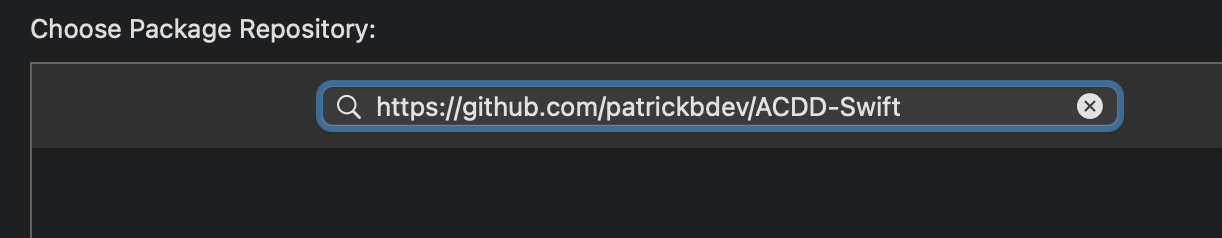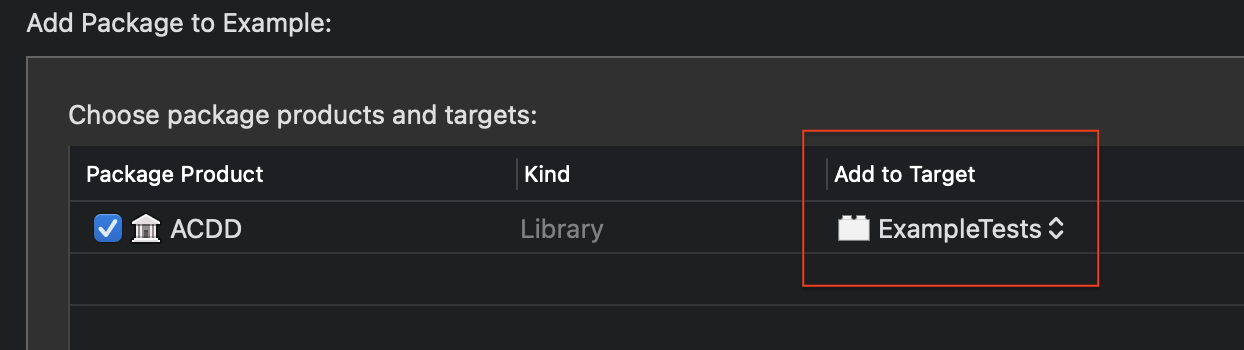# Swiftpack.co - ptrkstr/ACDD-Swift as Swift Package

Swiftpack.co is a collection of thousands of indexed Swift packages. Search packages.
ptrkstr/ACDD-Swift
Acceptance criteria driven development provides simple types for structuring scenario-oriented tests (given, when, then) to make following behavior-driven development in Swift easier.
.package(url: "https://github.com/ptrkstr/ACDD-Swift.git", from: "1.0.2")

# Acceptance Criteria Driven DevelopmentAcceptance criteria driven development provides simple types for structuring scenario-oriented tests (given, when, then) to make following behavior-driven development in Swift easier.

## Installation

### SPM

`https://github.com/ptrkstr/ACDD-Swift`1. Set the `Add to target` to your test target1. Import the module to a test file with:
`import ACDD`

## Usage

``````func test_success() throws {

var calculator = SumCalculator()

try AC(scenario: "Calculator should be able to sum")
.given("4 is entered into the calculator") {
calculator.firstNumber = 4
}
.and("5 is entered into the calculator") {
calculator.secondNumber = 5
}
.when("the sum is computed") {
try calculator.computeSum()
}
.then("the output is 9") {
XCTAssertEqual(calculator.output!, 9)
}
}
``````

### Logging

Logging is handled through the `LoggerType` protocol. You can create your own logger by adhering to this protocol and linking it using one of the below methods. This package comes with a `SimpleLogger` that prints to console like so:

``````AC - Calculator should be able to sum
- GIVEN 4 is entered into the calculator
- AND 5 is entered into the calculator
- WHEN the sum is computed
- THEN the output is 9
``````

#### Default Logger

Set the same logger for all `AC`s with:

``````ACDD.defaultLoger = SimpleLogger()
``````

This would be best placed in a location that runs before any tests. Suggestions in this SO post.

#### Per AC

Set a logger per `AC` with:

``````AC(scenario: "The world should not end", logger: HadronColliderLogger())
``````

## Notes

### Ordering

ACDD enforces ordering.

• The order is `given`, `when`, `then`.
• Any number of `and`s are allowed after each of the above clauses.
• How will you know if you've got the wrong order? The code won't compile 😉

### Omit handler

Every code handler is optional as there isn't always a need to write code. An example could be:

``````func test_no_input() throws {

var calculator = SumCalculator()

try AC()
.given("no numbers have been entered into the calculator")
.when("the sum is computed") {
XCTAssertThrowsError(try calculator.computeSum())
}
.then("an error occurs")
}
``````

### Omit given

Omitting `given` is allowed. This is helpful when applying BDD to UI development. Example:

``````func test_text_exists() throws {

var contentView: ContentView!

try AC()
.when("the screen appears") {
contentView = ContentView()
}
.then("I can see `Hello, world!`") {
let inspection = try contentView.inspect()
XCTAssertNoThrow(try inspection.find(text: "Hello, world!"))
}
}
``````

The above uses ViewInspector

### Exceptions

Every clause function rethrows a handler's error to remove the need to fill your tests with verbose `do {} catch {}`. If you want to opt into this, add:

• `try` before `AC`
• `throws` after your test function declaration

### GitHub

 link Stars: 1 Last commit: 2 weeks ago